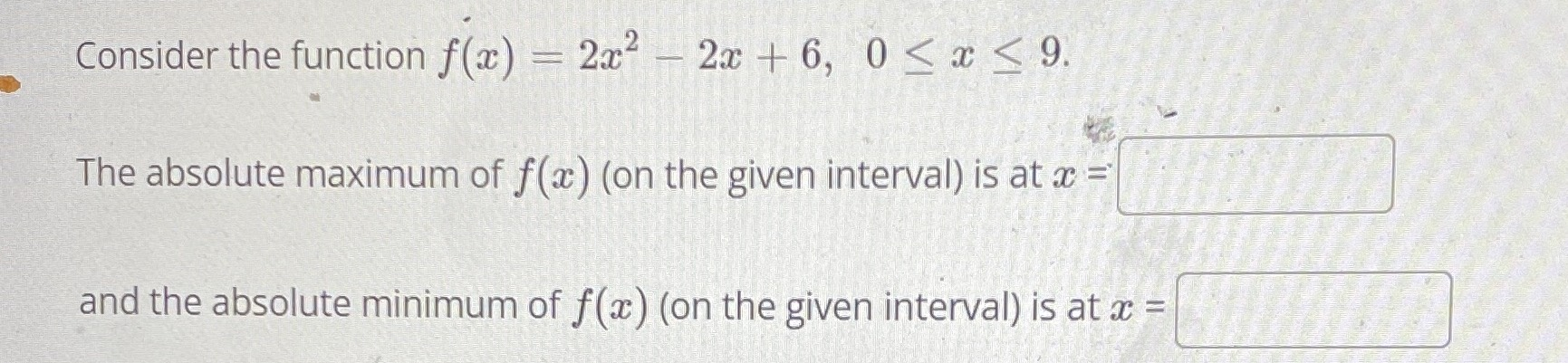### Still have math questions?

Algebra
QuestionConsider the function $$f ( x ) = 2 x ^ { 2 } - 2 x + 6,0 \leq x \leq 9$$

The absolute maximum of $$f ( x )$$ (on the given interval) is at $$x =$$ and the absolute minimum of $$f ( x )$$ (on the given interval) is at $$x =$$

minimum at x=$$\frac{1}{2}$$